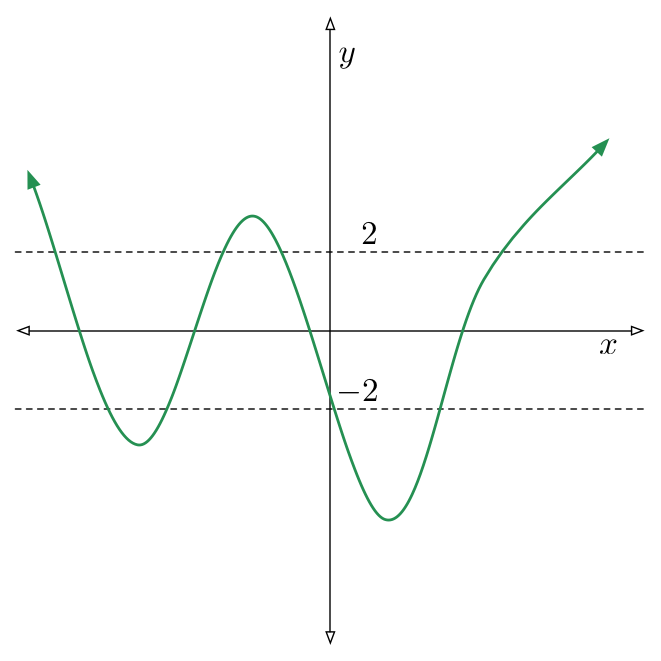# Why Isn't The Answer 10000?Consider all polynomials $f(x)$ with integer coefficients and degree at most 100. There are $N_f$ distinct integer values for which $f(n) = 2$, and $M_f$ distinct integer values for which $f(m)=-2$.

Over all such polynomials, what is the maximum possible value of $N_f \times M_f$?

×